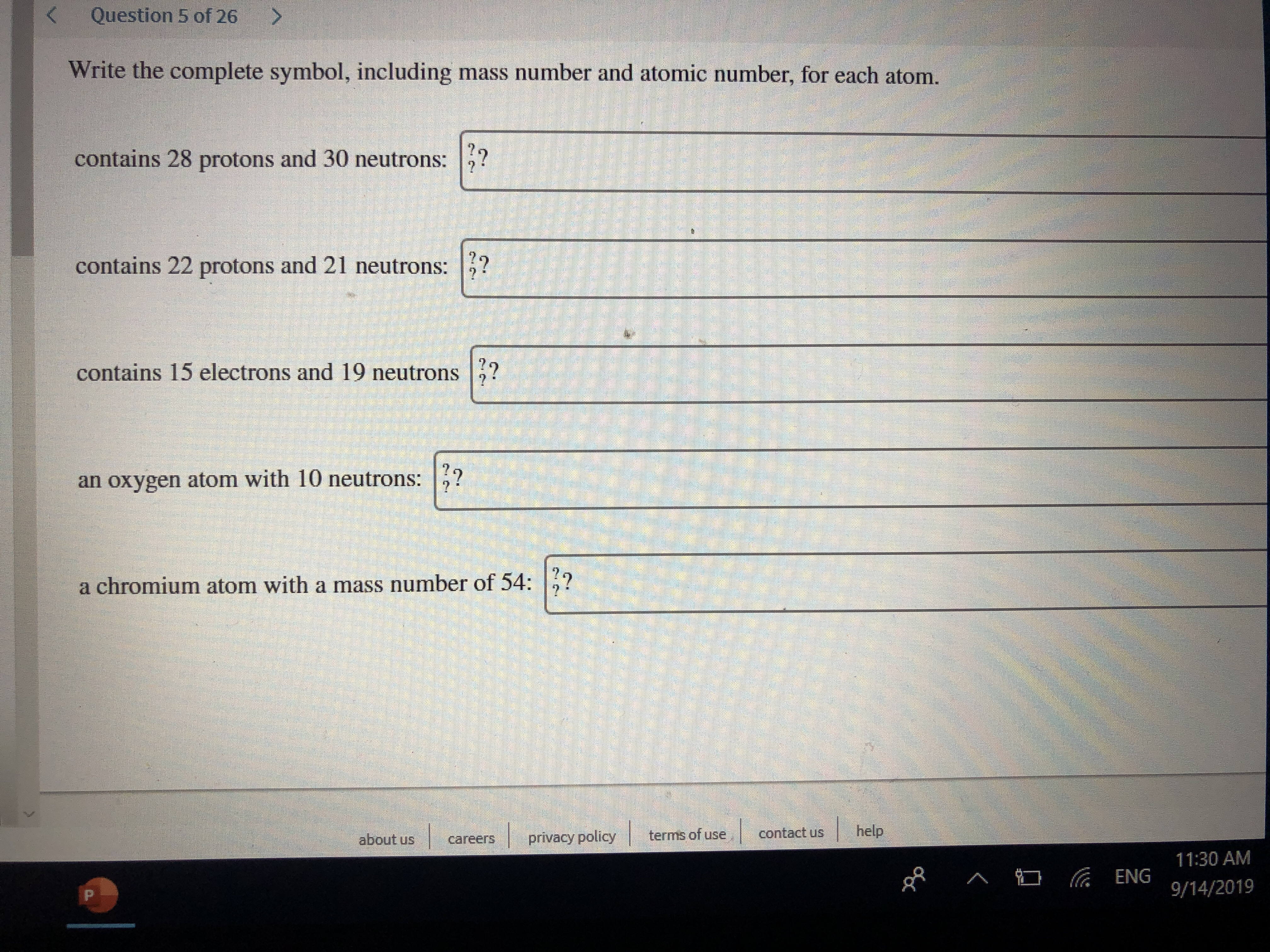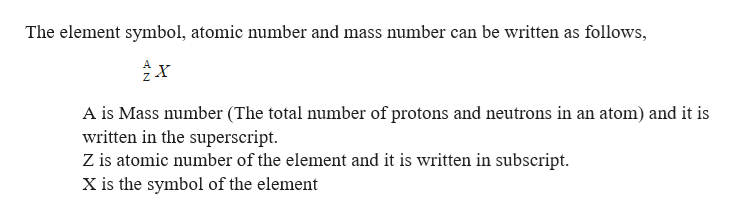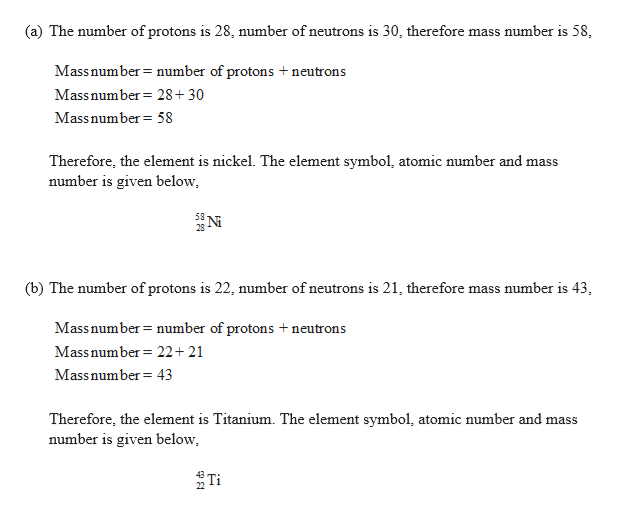# Question 5 of 26KWrite the complete symbol, including mass number and atomic number, for each atom.contains 28 protons and 30 neutrons:?contains 22 protons and 21 neutrons:contains 15 electrons and 19 neutronsan oxygen atom with 10 neutrons:a chromium atom with a mass number of 54:helpcontact usterms of useprivacy policyabout uscareers11:30 AMENG 9/14/2019P

Question
90 viewshelp_outlineImage TranscriptioncloseQuestion 5 of 26 K Write the complete symbol, including mass number and atomic number, for each atom. contains 28 protons and 30 neutrons:? contains 22 protons and 21 neutrons: contains 15 electrons and 19 neutrons an oxygen atom with 10 neutrons: a chromium atom with a mass number of 54: help contact us terms of use privacy policy about us careers 11:30 AM ENG 9/14/2019 P fullscreen
check_circle

Step 1

Symbol, mass number and atomic number has to be given.

Atomic mass:

The average atomic mass can be calculated by multiply the fraction by the mass number for each isotope and add together.help_outlineImage TranscriptioncloseThe element symbol, atomic number and mass number can be written as follows, A is Mass number (The total number of protons and neutrons in an atom) and it is written in the superscript Z is atomic number of the element and it is written in subscript X is the symbol of the element fullscreen
Step 2

Therefore, the element is n...help_outlineImage Transcriptionclose(a) The number of protons is 28, number of neutrons is 30, therefore mass number is 58 Mass number number of protons +neutrons Massnumber 28+30 Massnumber 58 Therefore, the element is nickel. The element symbol, atomic number and mass number is given below, 28 (b) The number of protons is 22, number of neutrons is 21, therefore mass number is 43 Mass number number of protons + neutrons Massnumber 22+ 21 Mass number 43 Therefore, the element is Titanium. The element symbol, atomic number and mass number is given below fullscreen

### Want to see the full answer?

See Solution

#### Want to see this answer and more?

Solutions are written by subject experts who are available 24/7. Questions are typically answered within 1 hour.*

See Solution
*Response times may vary by subject and question.
Tagged in

### General Chemistry